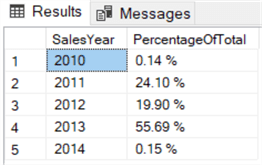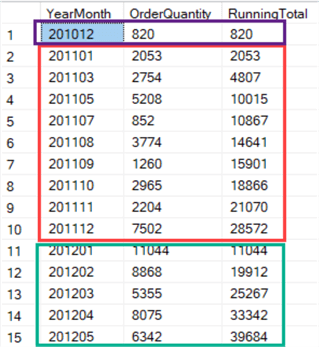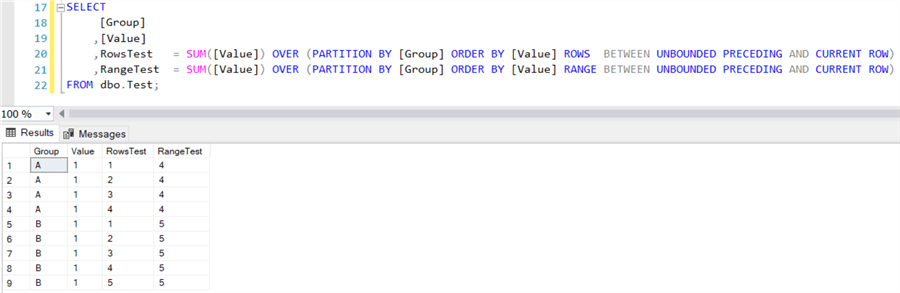# SQL Server Window Aggregate Functions SUM, MIN, MAX and AVG

##### Overview

In this part of the tutorial we’ll look at aggregate functions – sum, min, max, avg, etc. – and their relation with window functions.

##### Pre-2012 Support for Window Functions

Before the release of SQL Server 2012, there was already limited support for window functions. More specifically, since SQL Server 2005, you could already specify the OVER clause with a PARTITION BY clause, but the sorting and the frame extents were absent.

Let’s reprise our example from part 2, where we calculated the grand total of sales. We can add a similar calculation, but now with a PARTITION BY clause to retrieve the subtotal for each year:

```SELECT DISTINCT
SalesYear          = YEAR([OrderDate])
,SalesPerYear       = SUM([SalesAmount]) OVER (PARTITION BY YEAR([OrderDate]))
,SalesGrandTotal    = SUM([SalesAmount]) OVER ()
ORDER BY SalesYear;
```

This query can without a problem be executed on a SQL Server 2005 instance. By dividing the subtotal by the grand total, we can retrieve a percentage of total for each year:

```SELECT DISTINCT
SalesYear          = YEAR([OrderDate])
,PercentageOfTotal  = FORMAT(
SUM([SalesAmount]) OVER (PARTITION BY YEAR([OrderDate]))
/ SUM([SalesAmount]) OVER ()
,'P')
ORDER BY SalesYear;
```

Keep in mind that FORMAT is only available since SQL Server 2012. The result set:##### Aggregate Functions since SQL Server 2012

With the release of SQL Server 2012, full support was added for windowing with aggregate functions. Now we can add sorting and frame extents. This allows us to easily calculate a running total for example:

```WITH [CTE_source] AS
(
SELECT
[YearMonth]     = YEAR([OrderDate]) * 100 + MONTH([OrderDate])
,[Year]          = YEAR([OrderDate])
,[OrderQuantity] = SUM([OrderQuantity])
FROM [dbo].[FactResellerSales]
GROUP BY YEAR([OrderDate]) * 100 + MONTH([OrderDate]), YEAR([OrderDate])
)
SELECT
[YearMonth]
,[OrderQuantity]
,[RunningTotal] = SUM([OrderQuantity]) OVER (PARTITION BY [Year]
ORDER BY [YearMonth]
ROWS BETWEEN UNBOUNDED PRECEDING AND CURRENT ROW)
FROM [CTE_source]
ORDER BY [YearMonth];
```

By using PARTITION BY on the Year column, the running total is “reset” every January:The frame extent specifies “ROWS BETWEEN UNBOUNDED PRECEDING AND CURRENT ROW”. This means “the first row from the current window right until the current row”, where the first row is determined by the sort order. Also, because we sort by month, the running total is calculated correctly in chronological order. You can modify the query for other use cases, such as calculating a moving average: replace SUM with AVG and instead UNBOUNDED PRECEDING you choose the number of months to go back.

In earlier versions of SQL Server, calculating such a running total would require more complex queries which were not as performant as the window functions.

One important detail to keep in mind: once you specify an ORDER BY clause you’re in the post-2012 era of window functions. When you only specify a PARTITION BY clause, you’re in the 2005-era. If you specify an ORDER BY but not a frame extent, the default is used, which is RANGE BETWEEN UNBOUNDED PRECEDING AND CURRENT ROW. Let’s illustrate the effects with a simple example.

We have the following sample data:

```CREATE TABLE dbo.Test
(ID INT IDENTITY(1,1) PRIMARY KEY NOT NULL
,[Group] CHAR(1) NOT NULL
,Value INT NOT NULL);

INSERT INTO dbo.Test([Group],Value)
VALUES   ('A',1)
,('A',2)
,('A',3)
,('A',4)
,('B',5)
,('B',6)
,('B',7)
,('B',8)
,('B',9);
```

Let’s retrieve the maximum for each group with a window function with an ORDER BY specified:This did not go as expected. Because of the default frame extent, only the rows from the first row until the current row are considered for calculating the maximum. For the first row in group A, this is 1. For the second row, we have the values 1 and 2, so the maximum is 2. For the third row the maximum is 3 and so on. This issue can be solved in three ways:

• Don’t specify an ORDER BY clause, so we’re using the 2005-era window functions.
• Sort descending instead of ascending.
• Specify the frame extent ROWS BETWEEN UNBOUNDED PRECEDING AND UNBOUNDED FOLLOWING instead of using the default.
##### RANGE vs ROWS

As mentioned, the default frame extent uses RANGE instead of ROWS. But what is the difference between those two? The ROWS frame extent will limit the rows within the window by a fixed number of rows, ignoring the actual values in the rows. However, RANGE will also limit the rows but will look at the order by values to potentially include rows that have the same sorted values. Let’s illustrate by calculating running totals on our test table, but with all values equal to 1.In the case of ROWS, the running total is calculated correctly. However, when using RANGE, all rows within the partition are aggregated together, since they all have the same order by value as the current row. When you don’t specify a frame extent, RANGE will be used which can lead to incorrect results if rows have the same value. Furthermore, since RANGE has to inspect the rows for the actual values, performance is worse than when using ROWS.